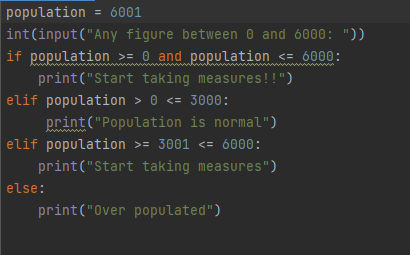# Python programm

Hello everyone.
I wrote this program with conditions but whenever I try to run it, it is printing out the same output for the conditions. What could be problem and how best can it be written?

``````population = 6001
int(input("Any figure between 0 and 6000: "))
if population >= 0 and population <= 6000:
print("Start taking measures!!")
elif population > 0 <= 3000:
print("Population is normal")
elif population >= 3001 <= 6000:
print("Start taking measures")
else:
print("Over populated")
``````I moved your question to its own topic because you were asking a question related to your own code for a challenge and were not answering the OP of the other thread. It is always best to create your own thread for you specific question(s).

I’ve edited your post for readability. When you enter a code block into a forum post, please precede it with a separate line of three backticks and follow it with a separate line of three backticks to make it easier to read.

You can also use the “preformatted text” tool in the editor (`</>`) to add backticks around text.

See this post to find the backtick on your keyboard.
Note: Backticks (`) are not single quotes (’).

can you explain what you want your program to do?

The propblem is population is always 6001, so ofcourse it’s always printing the same output.

1 Like

I am really not that experienced with Python, but in my mind it looks like a strange way to define what population intervals should be used. For example, how can you ever get the message in your first if-statement when the user only can give one input? (the user should never be able to give for example both 0 and 6000 at the same time, if you only accept one value, right?). I fiddled around with it based on similar reasoning with the intervals and wonder if this code performs the operations you intend (perhaps with a bit longer code):

``````population = int(input("Any figure between 0 and 6000: "))

if population == 0 or population == 6000:
print("Start taking measures!!")

elif population> 0 and population<=3000:
print("Population is normal")

elif population>= 3001 and population<6000:
print("Start taking measures")

elif population > 6000:
print("Over populated")
``````
1 Like

hello i think your (variable：population) is bigger than 6000. So your your program will only execute （else：）.Besides, your input is not assign to a variable. So your population is static. population’s value always 6001.you need code like this .

``````population = int(input("Any figure between 0 and 6000: "))
if 6000 >= population >= 0:
print("Start taking measures!!")
elif population > 0 <= 3000:
print("Population is normal")
elif population >= 3001 <= 6000:
print("Start taking measures")
else:
print("Over populated")
``````

in line 5 your indent is wrong!!!

you can just use else to consider value > 6000.i think use (>=) is ok.Change it is needless